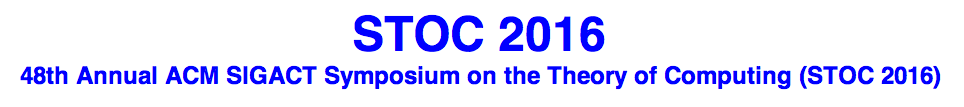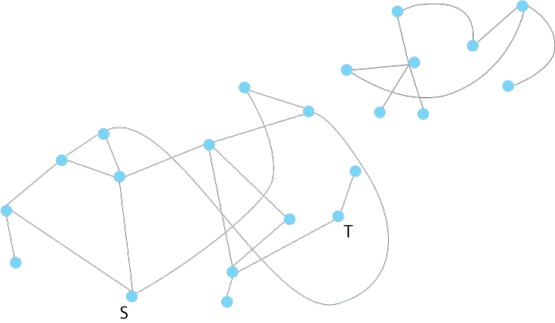# Columbia University computer scientists present three papers at STOC 2016Theoretical computer scientists from Columbia University are presenting three papers at this year’s ACM Symposium on the Theory of Computing (STOC) conference, held June 19 – 21. STOC is one of two annual flagship conferences (the IEEE Symposium on Foundations of Computer Science being the other) across all of theoretical computer science. Two papers being presented by Columbia researchers prove new lower bounds, showing that certain problems, one from circuit complexity and one from proof complexity, can’t be efficiently solved. The third paper, however, reports positive results and opens the door to solving certain dynamic graph problems using a deterministic rather than a randomized approach.

A key method of the two lower-bounds papers is random projections, an extension of the classic random restriction method in circuit complexity. Random restrictions are a well-studied way of “simplifying” circuits by randomly fixing some input variables to constants; these simplifications are useful in lower bound proofs. Random projections go one step further by identifying certain sets of variables in a careful way and setting them all to the same value (i.e., “projecting” a set of distinct input variables to a common new variable). These variable identifications enable a level of “control” over the simplification process that makes it possible to obtain sharper quantitative bounds.

#### Near-optimal small-depth lower bounds for small distance connectivity

Xi Chen, Columbia University
Igor C. Oliveira, Charles University in Prague
Rocco Servedio, Columbia University
Li-Yang Tan, Toyota Technological Institute at Chicago

Given a graph, does it contain a path of k or fewer edges between vertex s and vertex t? This is the well-studied “distance-k-connectivity” problem. It is well known that efficient algorithms can solve this problem on a standard sequential computer, but what if we want to use a highly parallel computer that has many processing elements that can run simultaneously, but only for an extremely small number of parallel computational steps?In Near-optimal small-depth lower bounds for small distance connectivity, the authors use the method of random projections to prove that any shallow circuit that solves the “small distance connectivity” problem must be very large. In the language of parallel computing, this translates into the following result: any highly parallel algorithm for this problem, running in very few parallel time steps, must use very many processors.

While previous results reached similar qualitative conclusions, this new paper gives significant quantitative improvements to those earlier lower bounds, providing a lower bound that almost matches known upper bounds.

Strengthening the paper’s lower bound to actually match the known upper bounds would imply a breakthrough in circuit complexity (since such a result would prove that the general undirected connectivity problem is not in the complexity class NC1).

#### Poly-logarithmic Frege depth lower bounds via an expander switching lemma

Toniann Pitassi, University of Toronto
Rocco Servedio, Columbia University
Benjamin Rossman, National Institute of Informatics, Tokyo
Li-Yang Tan, Toyota Technological Institute at Chicago

How difficult is it to prove theorems in a specific formal logic system?

One way to measure this difficulty is to give a lower bound on the length of the shortest proof, or on the minimal number of steps in a proof. Just as circuit complexity establishes upper and lower bounds on the size of circuits computing particular functions, proof complexity aims to give bounds on the length of proofs in specific formal proof systems, often borrowing methods used to prove lower circuit bounds. The paper Poly-logarithmic Frege depth lower bounds via an expander switching lemma falls into this framework.

The paper studies the complexity of proofs of a specific logical statement, the so-called “Tseitin principle.” This principle formalizes the well-known “handshake lemma” which states that the total number of handshakes experienced in any group of interacting people must be even no matter who shakes hands with whom (this is true simply because each individual handshake is experienced by two people). The paper studies these “Tseitin principles” on 3-regular expander graphs and investigates the complexity of “small-depth Frege proofs” of these principles on these graphs. Frege proofs are a strong proof system; previous efforts were able to show only that short proofs cannot exist for Tseitin principles if each line in the proof is only allowed to be a Boolean formula of very small depth. Using a new switching lemma for a carefully designed random restriction process over these expanders, this paper proves that short proofs cannot exist for Tseitin principles even if each line in the proof is allowed to be a much deeper Boolean formula.

#### Deterministic Decremental Single Source Shortest Paths: Beyond the O(mn) Bound

Aaron Bernstein, Columbia University
Shiri Chechik, Tel Aviv University

Given a large graph, what is the best way to recompute a shortest path when a single vertex or edge is removed? In an enormous graph, recomputing from scratch is no longer an option, and in the past 10-15 years computer scientists have found much faster ways to dynamically update graphs.

However, all such methods have been randomized (or incorporate randomization to some extent), where a small number of vertices and edges is sampled from an enormous graph to create a small, representative graph that can be more easily studied. Randomization helps ensure selected vertices or edges are representative of the whole graph; but it’s possible to get unlucky and randomly select vertices or edges that are not representative of the whole (just as a luckless pollster might randomly select all Democrats). It’s also possible—and especially problematic in graphs—that all selected edges or vertices are special in the same way (e.g., key servers in a network that handle large amounts of traffic). Such a lack of independence in the randomization could lead an adversary to delete exactly those vertices that were sampled.

With randomization seen as powerful but problematic, there has been a recent push to find a deterministic solution, with a couple of papers successfully incorporating deterministic aspects along with sampling.

In Deterministic Decremental Single Source Shortest Paths: Beyond the O(mn) Bound, Bernstein and Chechik describe the first wholly deterministic algorithm for computing a shortest path in the specific case when an edge is deleted and the path has to be reconstructed from a fixed source.

Rather than sampling, Bernstein and Chechik’s method stores information about every vertex and how it connects to its neighborhood. If an edge is removed, the stored information (which is not available to an adversary) is used to reconstruct what was there before.

This deterministic method is faster than recomputing from scratch, though it does not yet approach the speeds of randomized methods, which future work will address. More important for now, the method gives hope that other graph problems can be solved deterministically.

Posted 6/20/2016